## 编程珠玑-读书简记

2016年04月11日

### 开篇

A：我该如何对磁盘文件进行排序？
B：需要排序的内容是什么？文件中有多少条记录？每个记录的格式是什么？
A：该文件包含至多10,000,000个记录，每条记录都是一个7位整数。
B：如果文件那么小，为什么要使用磁盘排序呢？为什么不在主存中对它排序？
A：该功能是某大型系统中的一部分，大概只能提供1MB主存给它。
B：你能将记录方面的内容说得更详细一些吗？
A：每个记录是一个7位正整数，没有其它的关联数据，每个整数至多只能出现一次。
... ...


输入：

10秒钟是最适宜的运行时间。


### 啊哈！算法

给定一个包含32位整数的顺序文件，它至多只能包含40亿个这样的整数，并且整数的次序是随机的。请查找一个此文件中不存在的32位整数。 在有足够主存的情况下，你会如何解决这个问题？ 如果你可以使用若干外部临时文件，但可用主存却只有上百字节，你会如何解决这个问题？


请将一个具有n个元素的一维向量向左旋转i个位置。例如，假设n=8,i=3， 那么向量abcdefgh旋转之后得到向量defghabc。


reverse(0, i-1); // cbadefgh
reverse(i, n-1); // cbahgfed
reverse(0, n-1); // defghabc


给定一本英语单词词典，请找出所有的变位词集。例如，因为“pots”， “stop”，“tops”相互之间都是由另一个词的各个字母改变序列而构成的， 因此这些词相互之间就是变位词。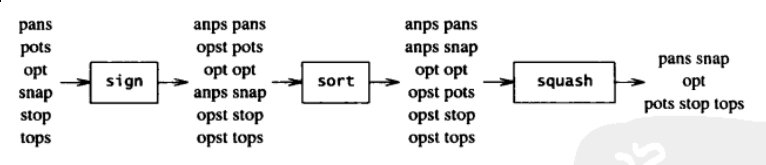### 数据决定程序结构

• 使用数组重新编写重复代码。冗长的相似代码常常可以使用最简单的数据结构—— 数组来更好地表述。

• 封装复杂结构。当需要非常复杂的数据结构时，使用抽象术语进行定义， 并将操作表示为类。

• 尽可能使用高级工具。超文本，名字-值对，电子表格，数据库， 编程语言等都是特定问题领域中的强大的工具。

• 从数据得出程序的结构。在动手编写代码之前，优秀的程序员会彻底理解输入， 输出和中间数据结构，并围绕这些结构创建程序。

### 编写正确的程序

• 使用断言。
• 顺序控制结构。
• 选择控制结构。
• 迭代控制机构。要证明循环的正确性就必须讨论三个性质：首先是初始化确立的循环不变式；其次是每次迭代保持不变式为真；最后是证明无论循环在何时终止执行，所得结果都是正确的。

### 编程中的次要问题

• 断言。
• 脚手架。
• 编码。对于比较难写的函数，最容易的方法是先用伪代码建立程序框架，然后翻译成要实现的语音。
• 测试。在脚手架中对组件进行测试比在大系统中要更容易彻底。

### 程序性能分析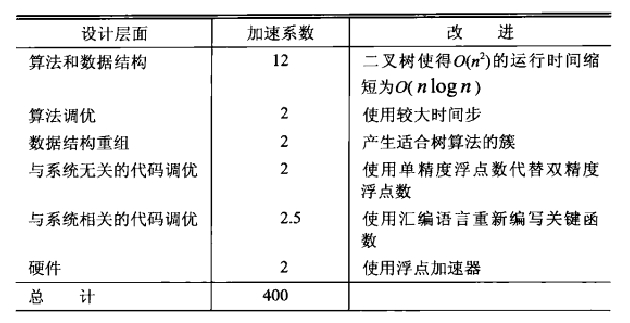1. 问题定义。良好的问题定义可以有效减少程序运行时间和程序长度。
2. 系统结构。将大型系统分解成模块，也许是决定其性能的最重要的单个因素。
3. 算法和数据结构。这个不用说了。
4. 代码调优。针对代码本身的改进。
5. 系统软件。有时候改变系统所基于的软件比改变系统本身更容易。
6. 硬件。更快的硬件可以提高系统的性能。

### 粗略估算

1英里 * 1/250英里 * 120英里/天  约等于  1/2 英里^3/天。


• 你需要把问题转换成一个可计算的具体模型。这一点往往不需要太担心，因为我们做的是估算，所以可以忽视很多无关紧要的因素，可以去简化你的模型， 记住我们要的只是一个粗略计算的结果。比如对于上面的问题，计算密西西比河一天流出多少水其实就是计算其一天的流量，利用中学所学知识， 流量 = 截面积 x 流速，那我们就只需计算密西西比河的出水口的截面积和流速即可。我们可以将出水口简化成一个矩形，因此就只需要知道出水口的宽和深即可。

• 你需要知道常识性的东西。上面我们已经把问题转换成了一个可计算的具体模型： 流量 = 出水口宽 x 出水口深 x 流速。接下来呢？你需要代入具体的数值去求得答案。而这就需要你具备一些常识性的知识了。比如作者就估计了密西西比河的出口有1英里宽， 20英尺深(如果你估计只有几十米宽，那就相差得太离谱了)。这些常识性的知识比第1点更值得关注，因为你无法给出一个靠谱的估算值往往是因为这点。

• 两个答案比一个答案好。即鼓励你从多个角度去对一个问题进行估算，如果从不同角度得到的答案差别都不大，说明这个估算值是比较靠谱的。

• 快速检验。即量纲检验。即等式两边最终的量纲要一致。 这一点在等式简单的时候相当显而易见。比如位移的单位是米，时间单位是秒， 速度单位是米/秒，那显然我们应该要用位移去除以时间来得到速度， 这样才能保证它们单位的一致。你可能会说，我了个去，这种小学生都懂的事， 你好意思拿出来讲。其实不然，当你面对的是一个具有多个变量的复杂物理公式，或者你提出某种物理假设，正在考虑将其公式化，该方法可以切切实实地帮你做出检验。

• 经验法则。“72法则”：1.假设以年利率r%投资一笔钱y年，如果r*y = 72， 那么你的投资差不多会翻倍。2.如果一个盘子里的菌群以每小时3%的速率增长， 那么其数量每天(24小时)都会翻倍。在误差不超过千分之五的情况下，$\pi$秒就是一个纳世纪。也就是说：3.14秒 = 10-9 * 100年 = 10-7 年。也就是说，1年大概是3.14x107 秒。所以如果有人告诉你，一个程序运行107 秒， 你应该能很快反应出，他说的其实是4个月。

• 实践。与许多其他活动一样，估算技巧只能通过实践来提高。

Little定律：系统中物体的平均数量等于物体离开系统的平均速率和每个物体在系统中停留 的平均时间的乘积。(如果物体离开和进入系统的总体出入流是平衡的， 那么离开速率也就是进入速率)。

### 算法设计技术

输入：



//第一个平方算法
maxsofar=0 for i = [0,n]
sum=0
for j = [i,n]
sum+=x[j]
maxsofar=max(maxsofar,sum)

//第二个平方算法 积分图类似的思想
cumarr[-1]=0 for i = [0,n]
cumarr[i]=cumarr[i-1]+x[i]
maxsofar=0for i = [0,n]
for j = [i,n]
sum=cumarr[j]-cumarr[i-1]
maxsofar=max(maxsofar,sum)


//分治思想 O(nlogn)
float recmax(int l, int u)
{   int i, m;
float lmax, rmax, sum;
if (l > u)  /* zero elements */
return 0;
if (l == u)  /* one element */
return max(0, x[l]);
m = (l+u) / 2;
/* find max crossing to left */
lmax = sum = 0;
for (i = m; i >= l; i--) {
sum += x[i];
if (sum > lmax)
lmax = sum;
}
/* find max crossing to right */
rmax = sum = 0;
for (i = m+1; i <= u; i++) {
sum += x[i];
if (sum > rmax)
rmax = sum;
}
return max(lmax + rmax,
max(recmax(l, m), recmax(m+1, u)));
}


//扫描算法int i;
float maxsofar = 0, maxendinghere = 0;
for (i = 0; i < n; i++)
{
maxendinghere += x[i];
maxendinghere = max(maxendinghere, 0);
maxsofar = max(maxsofar, maxendinghere);
}


• 保存状态，避免重复计算。
• 将信息预处理至数据结构中。
• 分治算法。
• 扫描算法
• 累积。
• 下界。本章例中为线性问题。

### 代码调优

• 不Van Wyk的图形程序。高效处理常见情况（热点）。
• 问题1——整数取模。利用等价的代数表达式，使用低开销的比较代替高的取模。
• 问题2——函数、宏和内联代码。通过宏替换函数来打破函数层次。
• 问题3——顺序搜索。使用哨兵来合并测试条件可以获得大约5%的提速。展开循环则可以56%的提速。
• 问题4——计算球面距离。修改数据结构，利用等价的代数表达式。
• 二分搜索——合并测试条件将每次内循环的比较次数从两次减为一次；利用等价的代数表达式将上下限的表示方法转换为下限与增量的表示法；展开循环。

so，just skip this chapter。

### 节省空间

• 不存储，重新计算。
• 稀疏数据结构。下面着重讲一下这点。
• 数据压缩。可以通过压缩的方式对对象进行编码，以减少存储空间。
• 分配策略。只有在需要的时候才进行分配。
• 垃圾回收。对废弃的存储空间进行回收再利用。

• 函数定义。用函数替换代码中的常见模式可以简化程序，同时减少代码的空间需求。
• 解释程序。用解释程序命令替换长的程序文本。
• 翻译成机器语言。可以将大型系统中的关键部分用汇编语言进行手工编码。

#### 稀疏数据结构#### 方法一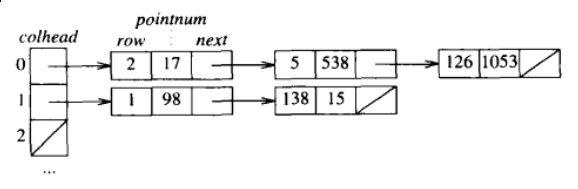#### 方法二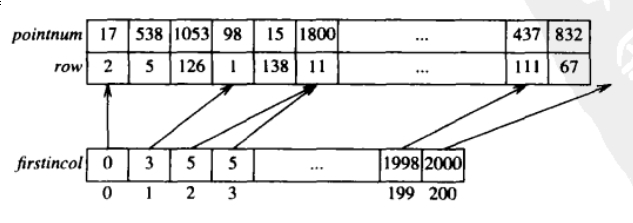firstincol是一个长度为201的数组，对于第i列，在数组row中， 下标为firstincol[i]到firstincol[i+1]-1对应的行元素非0，其值存储在相应的pointnum数组中。

### 排序

#### 插入排序

/* Simplest insertion sort */
void isort1()
{   int i, j;
for (i = 1; i < n; i++)
for (j = i; j > 0 && x[j-1] > x[j]; j--)
swap(j-1, j);
}

/* Write swap function inline */
void isort2()
{   int i, j;
DType t;
for (i = 1; i < n; i++)
for (j = i; j > 0 && x[j-1] > x[j]; j--) {
t = x[j];
x[j] = x[j-1];
x[j-1] = t;
}
}

/* Move assignments to and from t out of loop */
void isort3()
{   int i, j;
DType t;
for (i = 1; i < n; i++) {
t = x[i];
for (j = i; j > 0 && x[j-1] > t; j--)
x[j] = x[j-1];
x[j] = t;
}
}


#### 实现1：单向移动版本

/* Simplest version, Lomuto partitioning */
void qsort1(int l, int u)
{   int i, m;
if (l >= u)
return;
m = l;
for (i = l+1; i <= u; i++)
if (x[i] < x[l])
swap(++m, i);
swap(l, m);
qsort1(l, m-1);
qsort1(m+1, u);
}

/* Sedgewick's version of Lomuto, with sentinel 循环终止时，x[m]=t,用x[l]做哨兵省去了内循环中一次测试 */
void qsort2(int l, int u)
{   int i, m;
if (l >= u)
return;
m = i = u+1;
do {
do i--; while (x[i] < x[l]);
swap(--m, i);
} while (i > l);
qsort2(l, m-1);
qsort2(m+1, u);
}


#### 实现2：双向移动版本

小于等于pivot    ｜    ？    ｜    大于pivot
i            j


/* Two-way partitioning */
void qsort3(int l, int u)
{   int i, j;
DType t;
if (l >= u)
return;
t = x[l];
i = l;
j = u+1;
for (;;) {
do i++; while (i <= u && x[i] < t);
do j--; while (x[j] > t);
if (i > j)
break;
swap(i, j);
}
swap(l, j);
qsort3(l, j-1);
qsort3(j+1, u);
}


#### 进一步优化

if(l>=u)  改为  if(u-l > cutoff)


/* qsort3 + randomization + isort small subarrays + swap inline */
int cutoff = 50;
void qsort4(int l, int u)
{   int i, j;
DType t, temp;
if (u - l < cutoff)
return;
swap(l, randint(l, u));
t = x[l];
i = l;
j = u+1;
for (;;) {
do i++; while (i <= u && x[i] < t);
do j--; while (x[j] > t);
if (i > j)
break;
temp = x[i]; x[i] = x[j]; x[j] = temp;
}
swap(l, j);
qsort4(l, j-1);
qsort4(j+1, u);
}


### 取样问题

void GenKnuth(int m, int n) {
for(int i=0; i<n; ++i) {
if((bigrand()%(n-i)) < m) {
cout<<i<<endl;
--m;
}
}
}


void GenSets(int m, int n) {
set<int> s;
while(s.size() < m)
s.insert(bigrand() % n);
set<int>::iterator i;
for(i=s.begin(); i!=s.end(); ++i)
cout<<*i<<endl;
}


void GenShuf(int m, int n) {
int x[n];
for(int i=0; i<n; ++i)
x[i] = i;
for(int i=0; i<m; ++i) {
int j = randint(i, n-1);
swap(x[i], x[j]);
}
sort(x, x+m);
for(int i=0; i<m; ++i)
cout<<x[i]<<endl;
}

• 正确的理解所遇到的问题。
• 提炼出抽象问题。
• 考虑尽可能多的解法。很多程序员只愿花一分钟思考，花一天时间写代码，而不是花一个小时来思考，一个小时写代码。伪代码，抽象的数据类型。
• 实现一种解决方案。
• 回顾。经过充分的研究及思考，任何方案都可能被改进。

### 搜索

class IntSetArr {
private:
int n, *x;
public:
IntSetArr(int maxelements, int maxval)
{   x = new int[1 + maxelements];
n=0;
x = maxval; /* sentinel at x[n] */
}
int size() { return n; }
void insert(int t)
{   int i, j;
for (i = 0; x[i] < t; i++)
;
if (x[i] == t)
return;
for (j = n; j >= i; j--)
x[j+1] = x[j];
x[i] = t;
n++;
}
void report(int *v)
{   for (int i = 0; i < n; i++)
v[i] = x[i];
}
};


class IntSetList {
private:
int n;
struct node {
int val;
node *next;
node(int i, node *p) { val = i; next = p; }
};
node *head, *sentinel;
node *rinsert(node *p, int t)
{   if (p->val < t) {
p->next = rinsert(p->next, t);
} else if (p->val > t) {
p = new node(t, p);
n++;
}
return p;
}
public:
IntSetList(int maxelements, int maxval)
{   sentinel = head = new node(maxval, 0);
n = 0;
}
int size() { return n; }
void insert(int t) { head = rinsert(head, t); } //简单链表
void insert2(int t) //消除递归
{   node *p;
if (head->val == t)
return;
if (head->val > t) {
head = new node(t, head);
n++;
return;
}
for (p = head; p->next->val < t; p = p->next)
;
if (p->next->val == t)
return;
p->next = new node(t, p->next);
n++;
}
void insert3(int t) //两级指针
{   node **p;
for (p = &head; (*p)->val < t; p = &((*p)->next))
;
if ((*p)->val == t)
return;
*p = new node(t, *p);
n++;
}
void report(int *v)
{   int j = 0;
for (node *p = head; p != sentinel; p = p->next)
v[j++] = p->val;
}
};


class IntSetList2 {
private:
int n;
struct node {
int val;
node *next;
};
node *head, *sentinel, *freenode;
public:
IntSetList2(int maxelements, int maxval)
{   sentinel = head = new node;
sentinel->val = maxval;
freenode = new node[maxelements];
n = 0;
}
int size() { return n; }
void insert(int t)
{   node **p;
for (p = &head; (*p)->val < t; p = &((*p)->next))
;
if ((*p)->val == t)
return;
freenode->val = t;
freenode->next = *p;
*p = freenode++;
n++;
}
void report(int *v)
{   int j = 0;
for (node *p = head; p != sentinel; p = p->next)
v[j++] = p->val;
}
};


class IntSetBST {
private:
int n, *v, vn;
struct node {
int val;
node *left, *right;
node(int v) { val = v; left = right = 0; }
};
node *root;
node *rinsert(node *p, int t)
{   if (p == 0) {
p = new node(t);
n++;
} else if (t < p->val) {
p->left = rinsert(p->left, t);
} else if (t > p->val) {
p->right = rinsert(p->right, t);
} // do nothing if p->val == t
return p;
}
void traverse(node *p)
{   if (p == 0)
return;
traverse(p->left);
v[vn++] = p->val;
traverse(p->right);
}
public:
IntSetBST(int maxelements, int maxval) { root = 0; n = 0; }
int size() { return n; }
void insert(int t) { root = rinsert(root, t); }
void report(int *x) { v = x; vn = 0; traverse(root); }
};


class IntSetBST2 {
private:
int n, *v, vn;
struct node {
int val;
node *left, *right;
};
node *root, *freenode, *sentinel;
node *rinsert(node *p, int t)
{   if (p == sentinel) {
p = freenode++;
p->val = t;
p->left = p->right = sentinel;
n++;
} else if (t < p->val) {
p->left = rinsert(p->left, t);
} else if (t > p->val) {
p->right = rinsert(p->right, t);
} // do nothing if p->val == t
return p;
}
void traverse(node *p)
{   if (p == sentinel)
return;
traverse(p->left);
v[vn++] = p->val;
traverse(p->right);
}
public:
IntSetBST2(int maxelements, int maxval)
{   root = sentinel = new node;  // 0 if using insert1
n = 0;
freenode = new node[maxelements];
}
int size() { return n; }
void insert1(int t) { root = rinsert(root, t); }
void insert(int t)
{   sentinel->val = t;
node **p = &root;
while ((*p)->val != t)
if (t < (*p)->val)
p = &((*p)->left);
else
p = &((*p)->right);
if (*p == sentinel) {
*p = freenode++;
(*p)->val = t;
(*p)->left = (*p)->right = sentinel;
n++;
}
}
void report(int *x) { v = x; vn = 0; traverse(root); }
};



class IntSetBins {
private:
int n, bins, maxval;
struct node {
int val;
node *next;
node(int v, node *p) { val = v; next = p; }
};
node **bin, *sentinel;
node *rinsert(node *p, int t)
{   if (p->val < t) {
p->next = rinsert(p->next, t);
} else if (p->val > t) {
p = new node(t, p);
n++;
}
return p;
}
public:
IntSetBins(int maxelements, int pmaxval)
{   bins = maxelements;
maxval = pmaxval;
bin = new node*[bins];
sentinel = new node(maxval, 0);
for (int i = 0; i < bins; i++)
bin[i] = sentinel;
n = 0;
}
int size() { return n; }
void insert(int t)
{   int i = t / (1 + maxval/bins);  // CHECK !
bin[i] = rinsert(bin[i], t);
}
void report(int *v)
{   int j = 0;
for (int i = 0; i < bins; i++)
for (node *p = bin[i]; p != sentinel; p = p->next)
v[j++] = p->val;
}
};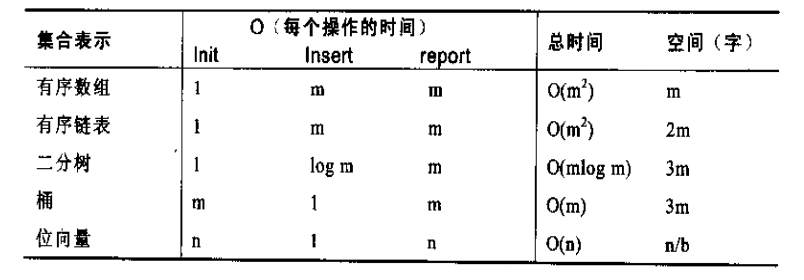• 库的作用。遇到涉及数据结构的问题时，第一反应应该是寻求解决问题的通用工具。当不满足时，专用的代码可以充分利用特定问题的性质，大大提高运行速度。
• 空间的重要性。
• 代码调优方法。最显著的是用只分配一个较大的内存块来替换通用内存分配。将递归函数重写为迭代可以使链表提速3倍，但只使箱提速10%。对大多数结构，引入哨兵可以获得清晰、简单的代码，缩短运行时间。

### 堆

// 最大堆实现, 数组下标从1开始，a不使用。

// 交换两数
void swap(int &a, int &b) {
int t = a;
a = b;
b = t;
}

// 把第i个元素向上移动
void ShiftUp(int a[], int i) {
while(i>1 && a[i]>a[i/2]) {
swap(a[i], a[i/2]);
i >>= 1;
}
}

// 把第i个元素向下移动
void ShiftDown(int a[], int n, int i) {
while((i=2*i) <= n) {
if(i+1<=n && a[i+1]>a[i]) ++i;
if(a[i] > a[i/2]) swap(a[i], a[i/2]);
else break;
}
}

// 把数组a变成具备最大堆性质的数组
void MakeHeap(int a[], int n) {
for(int i=n/2; i>0; --i)
ShiftDown(a, n, i);
}

// 向堆中插入元素x
void Insert(int a[], int &n, int x) {
a[++n] = x;
ShiftUp(a, n);
}

// 删除堆中第i个元素
void Del(int a[], int &n, int i) {
a[i] = a[n--];
if(i>1 && a[i]>a[i/2]) ShiftUp(a, i);
else ShiftDown(a, n, i);
}

// 堆排序，时间复杂度O(nlogn)
void HeapSort(int a[], int n) {
MakeHeap(a, n);
for(int i=n; i>1; --i) {
swap(a[i], a);
ShiftDown(a, i-1, 1);
}
}


### 字符串

int main(void) {
set<string> s;
set<string>::iterator j;
string t;
while(cin >> t)
s.insert(t);
for(j=s.begin(); j!=s.end(); ++j)
cout<<*j<<endl;
return 0;
}


int main(void) {
map<string, int> m;
map<string, int>::iterator j;
string t;
while(cin >> t)
m[t]++;
for(j=m.begin(); j!=m.end(); ++j)
cout<<j->first<<" "<<j->second<<endl;
return 0;
}


class Hash {
public:
Hash(): seed_(131), size_(0) {
memset(head_, 0, sizeof(head_));
}

void Insert(const char* str) {
unsigned int id = hash(str);
char *dst = (char*)node_[size_].word;
while(*dst++ = *str++);
node_[size_].next = head_[id];
head_[id] = &node_[size_];
++size_;
}

bool Find(const char* str) {
unsigned int id = hash(str);
for(Node* p=head_[id]; p; p=p->next) {
char* dst = (char*)p->word;
int i = 0;
for(; *(str+i) && *(str+i)==*(dst+i); ++i);
if(!*(str+i) && !*(dst+i)) return true;
}
return false;
}

private:
unsigned int hash(const char* str) {// BKDR Hash Function
unsigned int hash = 0;
while(*str) {
hash = hash * seed_ + (*str++);
}
return (hash & 0x7FFFFFFF) % kHashSize;
}

private:
unsigned int seed_;
unsigned int size_;
static const int kWordSize = 26 + 1;
static const int kNodeSize = 20000;
static const int kHashSize = 10001;
struct Node {
char word[kWordSize];
Node *next;
};
Node node_[kNodeSize];
Node* head_[kHashSize];
};


#### 后缀数组

char c = "hawstein";
char* pc;


for(int i=0; i<8; ++i)
pc[i] = &c[i];


sort(pc, pc+8, cmp);


inline bool cmp(char* p, char*q) {
return strcmp(p, q) < 0;
}


awstein
ein
hawstein
in
n
stein
tein
wstein


a
ana
anana
banana
na
nana


 许智磊，《后缀数组》。

 罗穗骞，《后缀数组——处理字符串的有力工具》。

#### 生成文本

/* markov.c -- generate random text from input document */

#include <stdio.h>
#include <stdlib.h>
#include <string.h>

char inputchars;
char *word;
int nword = 0;
int k = 2;

// 对word数组进行排序，得到指向同一个k单词序列的所有指针。int wordncmp(char *p, char* q)
{   int n = k;
for ( ; *p == *q; p++, q++)
if (*p == 0 && --n == 0)
return 0;
return *p - *q;
}

int sortcmp(char **p, char **q)
{   return wordncmp(*p, *q);
}

char *skip(char *p, int n)
{   for ( ; n > 0; p++)
if (*p == 0)
n--;
return p;
}

int main()
{
int i, wordsleft = 10000, l, m, u;
char *phrase, *p;
word = inputchars;

// 读入文件
while (scanf("%s", word[nword]) != EOF) {
word[nword+1] = word[nword] + strlen(word[nword]) + 1;
nword++;
}

// word数组后附加k个空字符（这样比较函数就不会运行到最后），并输出文档的前k个单词，然后调用排序
for (i = 0; i < k; i++)
word[nword][i] = 0;
for (i = 0; i < k; i++)
printf("%s\n", word[i]);
qsort(word, nword, sizeof(word), sortcmp);

// 二分搜索定位phrase的第一次出现，for循环扫描所有相同的词语，随机选择一个。如果该短语第k个单词的长度为0，则为文档最后一个，跳出循环。
phrase = inputchars;
for ( ; wordsleft > 0; wordsleft--) {
l = -1;
u = nword;
while (l+1 != u) {
m = (l + u) / 2;
if (wordncmp(word[m], phrase) < 0)
l = m;
else
u = m;
}
for (i = 0; wordncmp(phrase, word[u+i]) == 0; i++)
if (rand() % (i+1) == 0)
p = word[u+i];
phrase = skip(p, 1);
if (strlen(skip(phrase, k-1)) == 0)
break;
printf("%s\n", skip(phrase, k-1));
}
return 0;
}



/* markovhash.c -- generate random text, sped up with hash tables */

/* For storage efficiency (and also to minimize changes from markov.c),   the hash table is implemented in the integer array next.   If bin[i]=j, then word[j] is the first element in the list,   word[next[j]] is the next element, and so on. */

#include <stdio.h>
#include <stdlib.h>
#include <string.h>

char inputchars;
#define MAXWORDS 800000char *word[MAXWORDS];
int nword = 0;
int k = 2;

int next[MAXWORDS];
#define NHASH 499979int bin[NHASH];
#define MULT 31

unsigned int hash(char *ptr)
{   unsigned int h = 0;
unsigned char *p = ptr;
int n;
for (n = k; n > 0; p++) {
h = MULT * h + *p;
if (*p == 0)
n--;
}
return h % NHASH;
}

int wordncmp(char *p, char* q)
{   int n = k;
for ( ; *p == *q; p++, q++)
if (*p == 0 && --n == 0)
return 0;
return *p - *q;
}

int sortcmp(char **p, char **q)
{   return wordncmp(*p, *q);
}

char *skip(char *p, int n)
{   for ( ; n > 0; p++)
if (*p == 0)
n--;
return p;
}

int main()
{   int i, wordsleft = 10000, j;
char *phrase, *p;
word = inputchars;
while (scanf("%s", word[nword]) != EOF) {
word[nword+1] = word[nword] + strlen(word[nword]) + 1;
nword++;
}
for (i = 0; i < k; i++)
word[nword][i] = 0;
for (i = 0; i < NHASH; i++)
bin[i] = -1;
for (i = 0; i <= nword - k; i++) { /* check */
j = hash(word[i]);
next[i] = bin[j];
bin[j] = i;
}
for (i = 0; i < k; i++)
printf("%s\n", word[i]);
phrase = inputchars;
for ( ; wordsleft > 0; wordsleft--) {
i = 0;
for (j = bin[hash(phrase)]; j >= 0; j = next[j])
if ((wordncmp(phrase, word[j]) == 0)
&& (rand() % (++i) == 0))
p = word[j];
phrase = skip(p, 1);
if (strlen(skip(phrase, k-1)) == 0)
break;
printf("%s\n", skip(phrase, k-1));
}
return 0;
}


### 参考文献

 编程珠玑，第二版。

 Hawstein's Blog: 把《编程珠玑》读薄